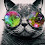## Sunday, August 30, 2015

### Cover Reveal: I Am Hope by Evelyn Shepherd #Excerpt #Giveaway

•=•=•=•=•=•=•=•=•=•=•=•=•=•=•=•=•=•=•=•=•=•=•=•=•=•=•=•=•=•

Evelyn Shepherd reveals the beautiful cover art for her next book in her THE METEORA TRILOGY series titled I AM HOPE.

•=•=•=•=•=•=•=•=•=•=•=•=•=•=•=•=•=•=•=•=•=•=•=•

BLURB

The undead were only the beginning.

Topher, Jesse, and Sawyer thought their greatest threat were the Infected and Mutated that roam the world. As they struggle to reach the camp in Kill Devil Hills in North Carolina, they soon discover that the monstrous mutations of the undead are the least of their problems. Something else, something far more intelligent and lethal, is hunting them.

Topher knows that the only way humanity will survive is to find a cure for the strange infection that’s ravaged the world. He’s on the brink of discovering it; but the further his research goes, the more he realizes that curing the world of the infection may mean losing Sawyer.

The three will fight to stay together, and Topher will have to make the ultimate choice: cure the world or save his lover?

•=•=•=•=•=•=•=•=•=•=•=•=•=•=•=•=•=•=•=•=•=•=•=•=•=•=•=•=•=•

PRESENTING

Cover Art by Valerie Tibbs

•=•=•=•=•=•=•=•=•=•=•=•=•=•=•=•=•=•=•=•=•=•=•=•

EXCLUSIVE EXCERPT

Sawyer nodded, his hands clamped between his knees. His head lined up perfectly with my shoulder, so he could comfortably rest it against me. The first time I’d seen him, my heart had stopped. Sawyer had been the snarky punk in my ex-lover’s SUV. I should have been jealous, but all I had wanted was to see him smile.

I pressed a kiss to Sawyer’s forehead. “How are you doing?”

“I’m not the one who got shot.”

“True, but a lot has been happening lately. Between Salvation, the farm, and now this…it’s a lot to take in.” I reached down and took his hand in mine, pressing our palms together. His fingers danced over mine, tracing patterns on the back of my hand and coming around to write letters on my palm.
“Do you think the world is worth saving?” Sawyer asked.

Read more exclusive excerpts on –

•=•=•=•=•=•=•=•=•=•=•=•=•=•=•=•=•=•=•=•=•=•=•=•

Evelyn Shepherd lives in Columbus, Ohio with two fat cats. Her time is split between writing and running a book/writing blog. She’s the author of the Theo Bourne Series, the best-selling Last Canticle, and the award-winning Meteora Trilogy.

||  Blog & Website  ||  Facebook Page  ||  Twitter  ||  Tumblr  ||  Email  ||

•=•=•=•=•=•=•=•=•=•=•=•=•=•=•=•=•=•=•=•=•=•=•=•

RAFFLECOPTER GIVEAWAY

Winner’s Prize: E-copies of I AM HERE (Book 1) and I AM ALIVE (Book 2)

#### 1 comment:

1.This comment has been removed by the author.